#### Michael Brachmann### 2. Vizier isn't Just Code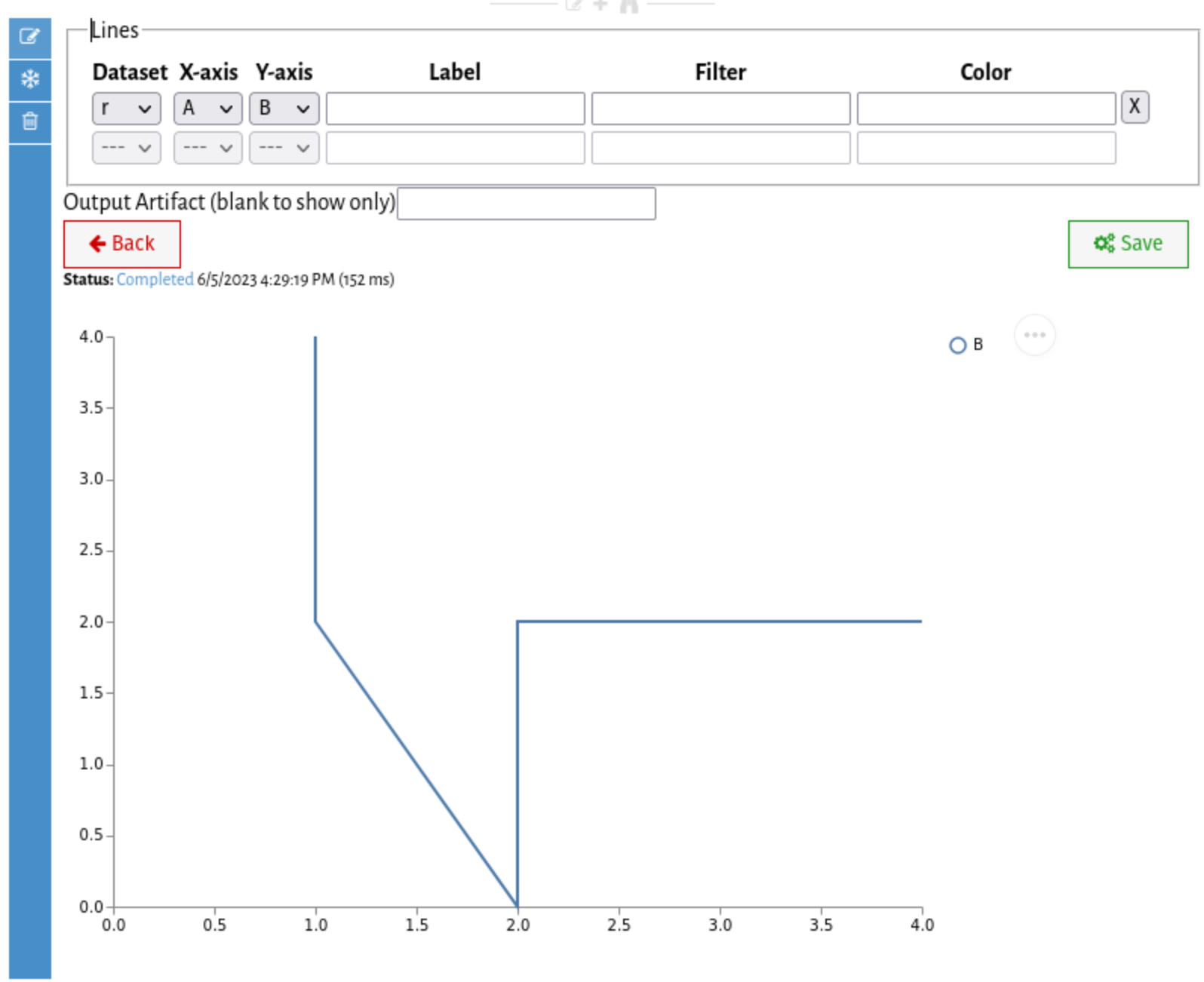### Including...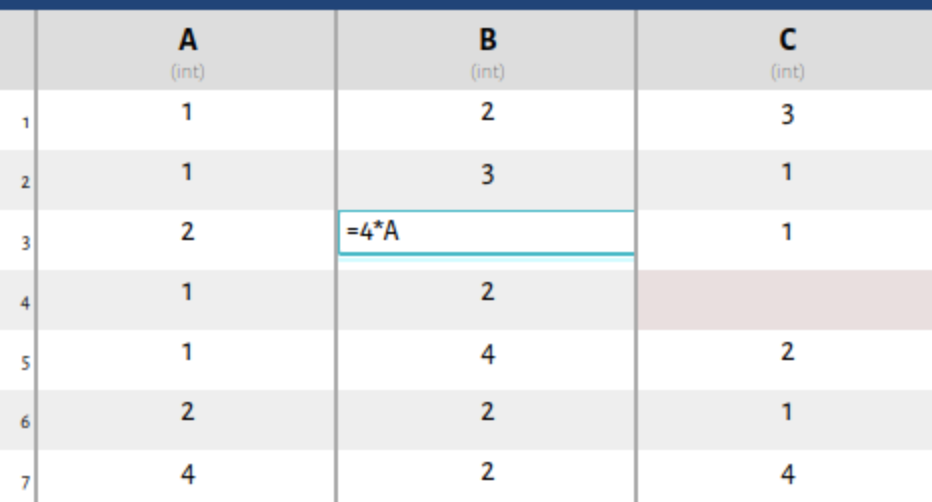(Wrangler: Interactive Visual Specification of Data Transformation Scripts; Kandel et al; CHI 2011)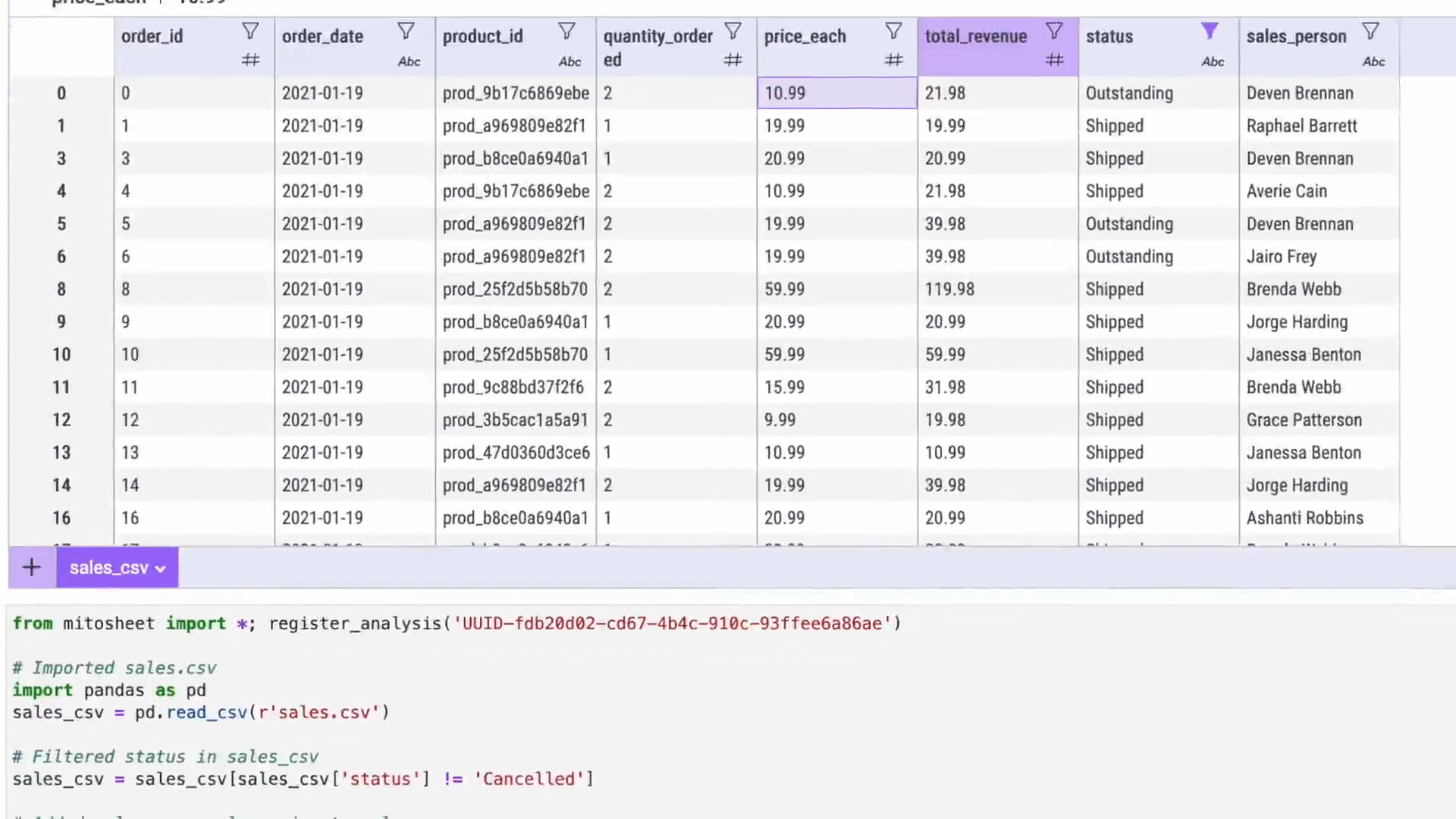(trymito.io)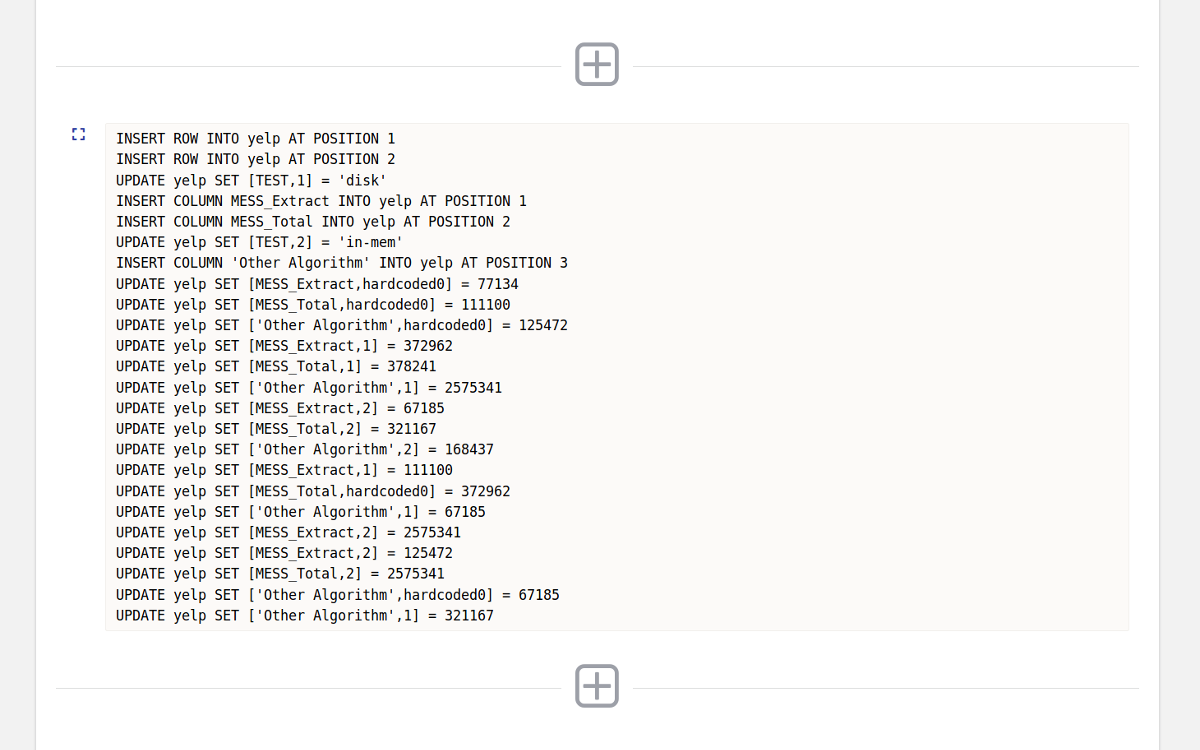(The Exception that Improves the Rule; Freire et. al.; HILDA 2016)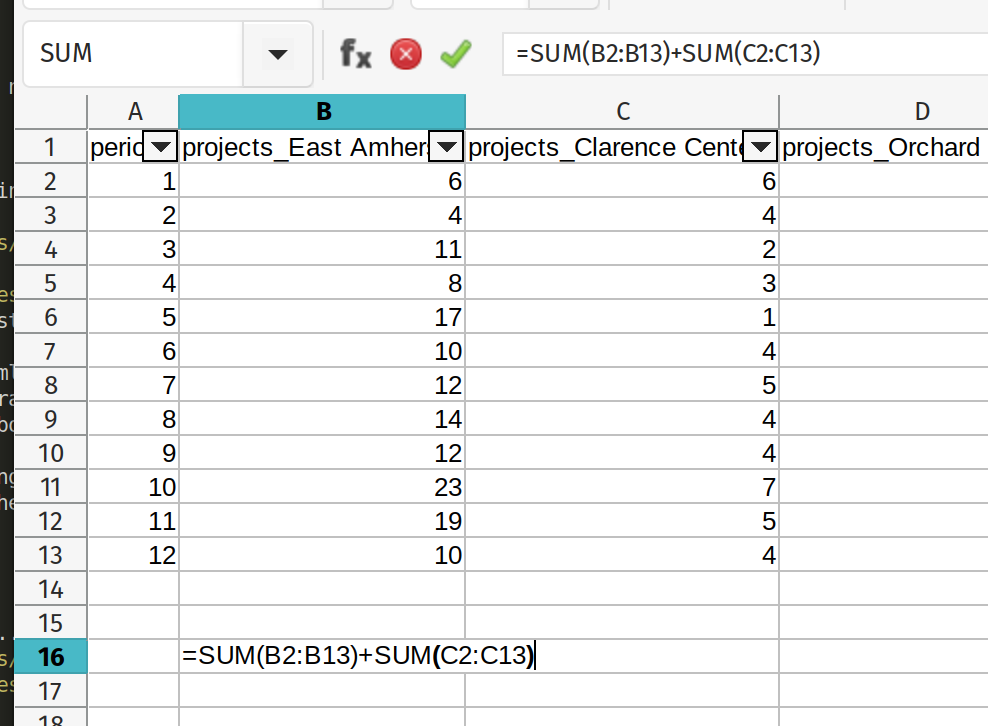### Goals

• ... that can replay edits on a new dataset.
• ... while supporting "free-form" editing.

### Shape Changes

#### Mapping Functions

$row(n) = \begin{cases} n & \textbf{if } \color{#d40000}{\blacksquare}, \color{#6c5353}{\blacksquare}\\ n+1 & \textbf{if } \color{#008080}{\blacksquare}, \color{#a05a2c}{\blacksquare}\\ n-3 & \textbf{if } \color{#808000}{\blacksquare} \end{cases}$          $col(n) = \begin{cases} n & \textbf{if } \color{#d40000}{\blacksquare}, \color{#008080}{\blacksquare}\\ n-1 & \textbf{if } \color{#6c5353}{\blacksquare}, \color{#a05a2c}{\blacksquare}\\ \texttt{null} & \textbf{if } \color{#536c53}{\blacksquare}\\ \end{cases}$

### Coping with Big Data

2. DataSpread (Bendre et. al.; ICDE 2018)
3. Ignore the Problem (This Talk)

### Coping with Big Data

Only materialize the subset of visible rows
(and/or columns)

### Restricting the Overlay

We need to materialize the
transitive closure
of the cell dependencies.

### Restricting the Overlay

Spreadsheets are optimized for reactive, interactive computation over cells.

... but here we have a big bulk computation.

### Recursive Patterns

$$H = G$$ $$H[n] = G[n] + H[n-1]$$
↓ $$H[n] = sum(G[0:n])$$

Many common patterns have an
equivalent closed-form representation.

### Recursive Patterns

$$H[0:1] = G[0:1]$$ $$H[n] = G[n] + H[n-2]$$

SELECT G + lag(H, 2) AS H OVER (ORDER BY row) FROM ...


Window queries work for the rest.

### Recursive Patternshttps://vizierdb.info